Latest Banking jobs   »   Quantitative Aptitude Quiz For RRB PO...

# Quantitative Aptitude Quiz For RRB PO Mains 2021- 22nd September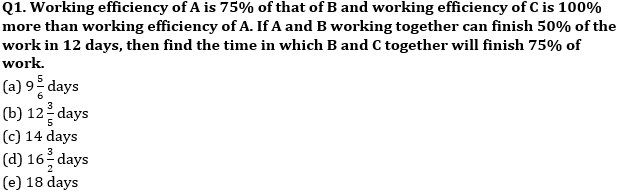Q2. If sum of circumference of a circle and perimeter of rectangle is 204 cm and perimeter of rectangle is 50% of magnitude of area of square and side of square is 12 cm .If length of rectangle is 8 cm more than that of breadth, then find sum of area of circle and rectangle ?
(a) 1694sq.cm
(b) 1450sq cm
(c) 1550sq cm
(d) 1750sq cm
(e) 1460sq cm

Q3. A started a business with Rs 9000 and B joined him with Rs 16000 after 3 months. After a year ‘A’ got 35% of total profit for his managerial work while remaining profit is divided into A and B according to their investment. If after an year ‘A’ got Rs 52800 as his share, the total profit earned by them?
(a) 74,000
(b) 76,000
(c) 80,000
(d) 84,000
(e) 88,000

Q4. In vessel A 80% is milk and rest is water. Vessel B also contain same mixture in which milk is 62½% of total volume. 25ℓ of mixture taken out from vessel A and poured in vessel B so that milk becomes 100% more than water in vessel B. If new quantity of mixture in vessel B is 16% less than initial quantity of mixture in vessel A then find initial quantity of milk in vessel A ?
(a) 100l
(b) 125l
(c) 115l
(d) 105l
(e) 112l

Q5. A man invests Rs. 1,200 at 10% p.a. At the end of the year he withdraws 30% of total amount and pays Rs. 24 as transaction fee. At the end of 2nd year he withdraws 30% of the amount and pays Rs. 93 as transaction fee. What is the balance at the end of the third year?
(a) Rs. 660
(b) Rs. 825
(c) Rs. 500
(d) Rs. 770
(e) None of these

Q6. Two type of Rice are mixed in ratio 3 : 2. If the price of rice is in the ratio 8 : 9 and mixture is sold at 20% profit then find the price of each type of Rice. Given that mixture was sold Rs. 40.32 per kg.
(a) 32 Rs. & 36 Rs.
(b) 36 Rs. & 40.5 Rs.
(c) 24 Rs. & 27 Rs.
(d) 28 Rs. & 31.5 Rs.
(e) None of these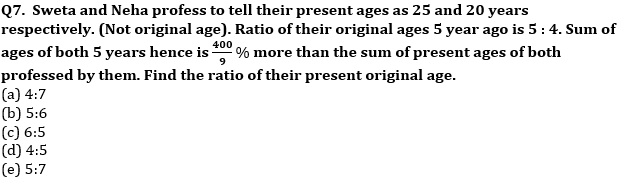Q8. Satish borrow education loan of Rs 75000 from SBI at the rate of 10% compounded annually, if after 2 year he paid an amount of 30750, then find how much more amount Satish have to pay to clear his loan in next 2 years?
(a) Rs 76200
(b) Rs 72600
(c) Rs 70600
(d) Rs 72000
(e) Rs 72300

Q9. A shopkeeper marked up the price of a mobile phone by 40% of its cost price, if he increases the discount from 5% to 10%, the profit would decrease by 1400 Rs. How much profit shopkeeper would earn if he gives a discount of 20% on the marked price ? Assume that, he calculates discount only on MRP.
(a) 1800 Rs.
(b) 1200 Rs.
(c) 2800 Rs.
(d) 2200 Rs.
(e) 2400 Rs.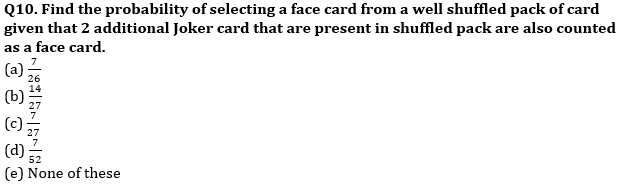Solutions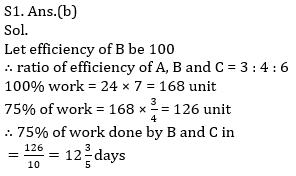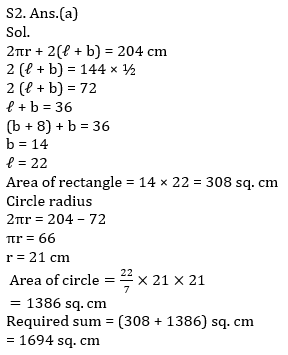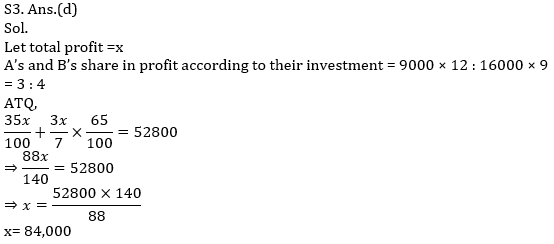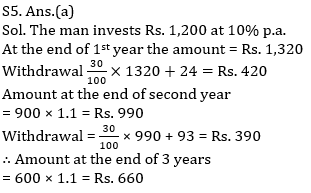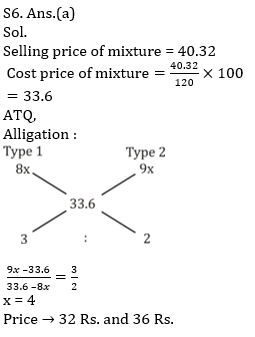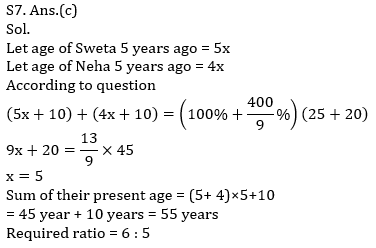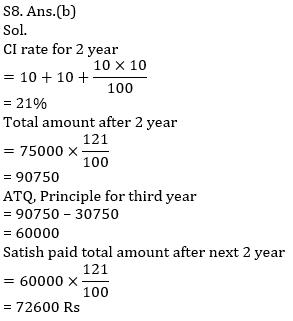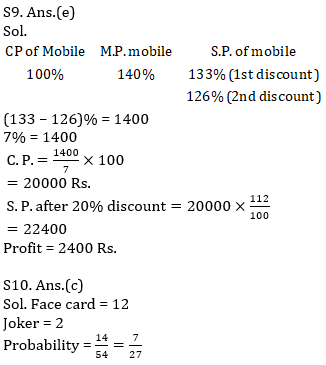Click Here to Register for Bank Exams 2021 Preparation Material#### Congratulations!Download Hindu Review of October 2021: Free PDF